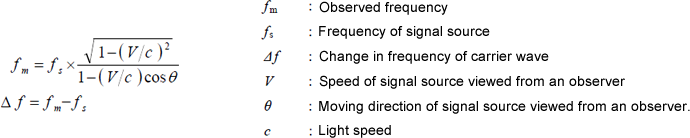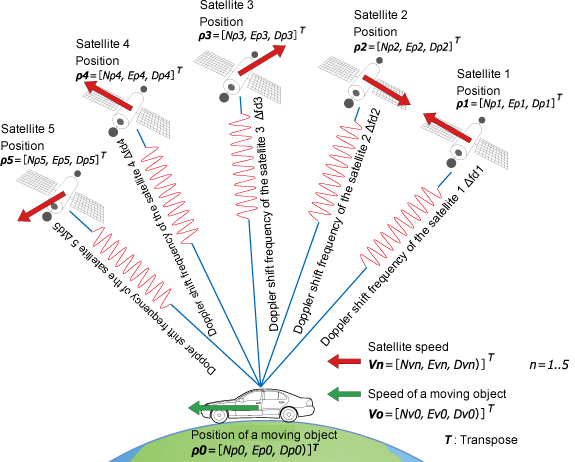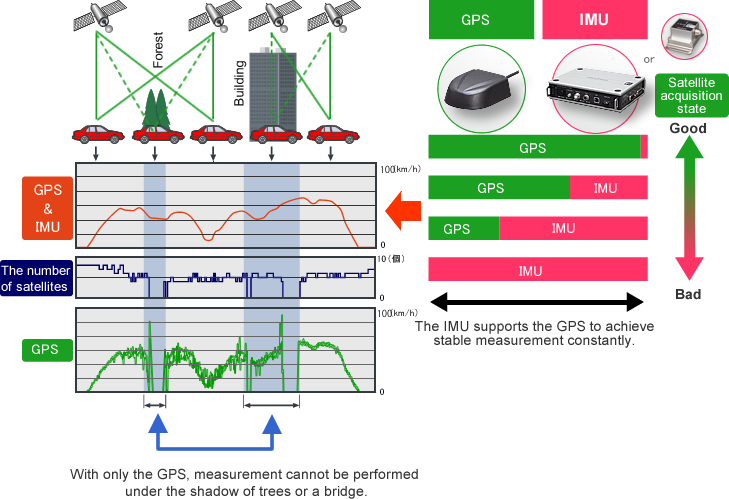Principle of speed measurement using GPS

LC-8000series

Principle of speed measurement using GPS

The LC-8310 compact & high-sensitive GPS speedometer calculates speed by using Doppler effect of the carrier waveform from GPS satellites.

The Doppler effect is the phenomena that is observed whenever the source of waves such as radio wave, light wave, or sound wave is moving with respect to an observer. The frequency will be high when the observer is approaching to the source, and low frequency when the source is receding.

The following formulas show the Doppler effect of the radio wave and light wave.Carrier wave frequency of GPS satellites (L1 band fs=1.5754 GHz) is controlled very precisely. Measuring the frequency and applying it to the above formula derives the speed V.
However, the ground speed cannot be measured by the carrier wave frequency of only one GPS satellite because GPS satellite is moving at high speed, and also has the effect of the rotation of the earth. Therefore the LC-8310 uses carrier waves from multiple GPS satellites (more than four) to measure the frequency as shown below, and then calculates the speed of the moving object by the same way of positioning measurement method.In this method, the speed of the moving object can be calculated extremely more precisely than the calculation method using the amount of position change, because it receives almost none of the influence from the ionosphere.

The accuracy of its horizontal component is 0.003 m/s when 2 σ, 0.08 m/s when 3 σ. However, it shall not apply to the accuracy of the vertical components. As for the accuracy of the vertical component, the accuracy turns worse approx. 3 times in comparison with the accuracy of the horizontal components because it receives a satellite signal from only a single direction. From the image above, you can see that the moving object is receiving the radio waves of various Doppler shift frequencies from multiple satellites.

The formulation of these relationships is as follows.

Δ fd1 = 1/ λ (V0 - V1)· u1
Δ fd2 = 1/ λ (V0 - V2)· u2
Δ fd3 = 1/ λ (V0 - V3)· u3
Δ fd4 = 1/ λ (V0 - V4)· u4
Δ fd5 = 1/ λ (V0 - V5)· u5

λ is wavelength of carrier waveform radiated from a satellite; approx. 0.19m, fb represents clock error of a receiver; (Hz), and un (n = 1..5)shows visual direction unit vector of a satellite and a receiver position. Those can be calculated by the following formulas.

un =ρ n/| ρ n| (n = 1..5)
ρ n =［ (Npn - Np0), ( Epn - Ep0), ( Dpn - Dp0)］T (n = 1..5)

The speed of a moving object can be calculated by solving Vo with these formulas.

Stable measurement
– Correction processing by IMU –

A big noise is occurred in speed output of the Doppler method on a public road in city area. Because there are enough obstacles to give the influence of multi-path on the measurement such as trees or buildings as shown below. In addition, the speed measurement using GPS alone cannot be performed when the vehicle passes the place that satellite signal cannot be captured, like the viaduct.

Even under the multi-path environment, combining the speed information from GPS and tri-axial accelerometers of IMU/tri-axial gyro with appropriate ratio derives more precise and much smoother speed data output than that of one GPS as is shown below.

Also, interpolation by IMU helps high accurate speed data output at the place where the measurement in GPS alone is not possible in the cases such that a vehicle passes under a viaduct.Revised:2019/3/20